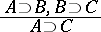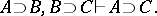# Derived rule

(diff) ← Older revision | Latest revision (diff) | Newer revision → (diff)

of a derivation in a given calculus

A derivation rule whose conclusion is derivable from its premises in the calculus under consideration. For example, in propositional calculus the derivation ruleis a derived rule, since in this calculus there is derivability from the premises:Every derived rule is a sound rule, but not every sound rule is a derived rule. For example, the substitution rule in propositional calculus is a sound but not a derived rule.

How to Cite This Entry:
Derived rule. Encyclopedia of Mathematics. URL: http://encyclopediaofmath.org/index.php?title=Derived_rule&oldid=15149
This article was adapted from an original article by S.N. Artemov (originator), which appeared in Encyclopedia of Mathematics - ISBN 1402006098. See original article